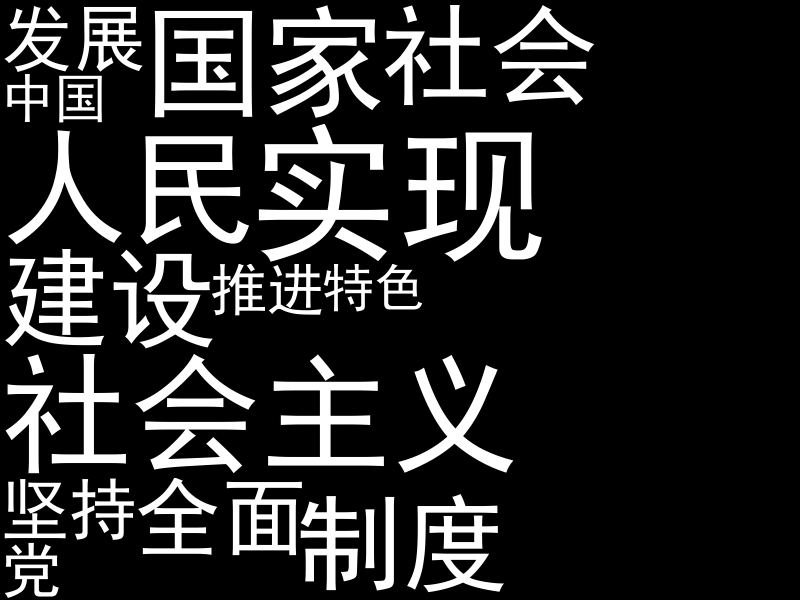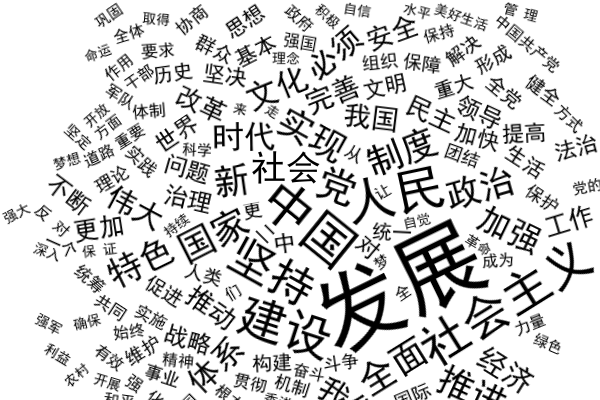### Wordcloud 探秘

1. 它使用了 Pillow 库，搜索发现这是一个 python 图像处理库。
2. 它在一个 `for word, freq in frequencies` 循环中，将单词逐个放到图片上。
3. 这个循环中，除了对字体进行设置外，还调用了一个 sample_position 方法来寻找放置的位置。<- 这就是我们所要的
4. sample_position 很简单，它的实现在 query_integral_image.pyx 文件中，仅有 30 多行，它从图片左上角第一个像素开始，遍历所有像素，检查各个位置能否放置。另外，integral_image 翻译为 积分图。

### 词云布局方法

wordcloud 库使用了一个非常简单的布局方法：

1. 从左上角第一个像素开始，判断在这个位置放置新词语会不会与已放置的内容重叠；
2. 如果不重叠，将这个位置添加一个列表 list 中；
3. 遍历图片所有像素，将所有可放置的位置都加到 list 中；
4. 从 list 中随机选择一个位置放置这个词语。

1. 选择一个初始位置 P；
2. 对每一个单词，从位置 P 开始，稍微移动一点点距离，看能不能放下，如果不能，再稍微移动一点距离，直到能够放下。

### 着手实现

``````import random
import math

from PIL import Image
from PIL import ImageDraw
from PIL import ImageFont

words = ['发展', '中国', '人民', '建设', '社会主义', '坚持', '党', '全面', '国家', '实现', '制度', '推进', '特色', '社会']

# 创建一张 800×600 的图片
img = Image.new("L", (800, 600))
draw = ImageDraw.Draw(img)

for word in words:
# 选择字体和大小：黑体，大小随机
font_size = random.randint(50,150)
font = ImageFont.truetype('C:\Windows\Fonts\SIMHEI.TTF', font_size)

# 计算文字矩形框的大小
box_size = draw.textsize(word, font=font)

# 在图片中寻找一个能放下矩形框的位置
x, y = find_position(img, box_size, box_size)
if x:
# 找到一个位置，并绘制上文字
draw.text((x, y), word, fill="white", font=font)
img.show()
``````

``````import numpy as np
def find_position(img, size_x, size_y):
# 计算积分图，cumsum([1,2,3,4]) => [1,3,6,10]
integral = np.cumsum(np.cumsum(np.asarray(img), axis=1), axis=0)

# 使用 wordcloud 的布局策略
for x in range(1, 800 - size_x):
for y in range(1, 600 - size_y):
# 检测矩形区域内容是否为空
area = integral[y - 1, x - 1] + integral[y + size_y - 1, x + size_x - 1]
area -= integral[y - 1, x + size_x - 1] + integral[y + size_y - 1, x - 1]
if not area:
return x, y

return None, None
``````### 明显的缺点

wordcloud 使用了很多使代码变得难以理解的优化策略，并且将 find_position 函数转用 Cython 实现（转换为 C 语言）也在很大程度上说明了这个问题。

### 四叉树

``````class QuadTree:
x1 = 0
y1 = 0
x2 = 0
y2 = 0
children = None

def __init__(self, x1, y1, x2, y2):
self.x1,self.y1,self.x2,self.y2 = x1,y1,x2,y2

# 计算积分图
integral = np.cumsum(np.cumsum(np.asarray(sprite.img), axis=1), axis=0)

def build_tree_recursive(x1, y1, x2, y2):
area = integral[y1 - 1, x1 - 1] + integral[y2, x2]
area -= integral[y1 - 1, x2] + integral[y2, x - 1]
if not area:
# 区域内没有像素
return None

# 区域内有像素，继续划分
children = []
cx = int((x1 + x2) / 2)
cy = int((y1 + y2) / 2)

# 最小矩形区域边长为 1 像素
if x2 - x1 > 1 or y2 - y1 > 1:
c0 = build_tree_recursive(x1, y1, cx, cy)  # 左上区域
c1 = build_tree_recursive(cx,  y1, x2, cy) # 右上区域
c2 = build_tree_recursive(x1, cy, cx, y2)  # 左下区域
c3 = build_tree_recursive(cx, cy, x2, y2)  # 右下区域
if c0:
children.append(c0)
if c1:
children.append(c1)
if c2:
children.append(c2)
if c3:
children.append(c3)
if len(children):
tree.children = children
return tree
``````

### 四叉树重叠检测

``````# (x1,y1) 为 tree 对应单词放置位置，(x2,y2) 为 otherTree 对应单词放置位置
def overlaps(tree, other_tree, x1, y1, x2, y2):
if rectCollide(tree, other_tree, x1, y1, x2, y2):
if not tree.children:
if not other_tree.children:
return True
else:
for i in range(0, len(other_tree.children)):
if overlaps(tree, other_tree.children[i], x1, y1, x2, y2):
return True
else:
for i in range(0, len(tree.children)):
if overlaps(other_tree, tree.children[i], x2, y2, x1, y1):
return True
return False

# 位于 (x1,y1) 的四叉树中矩形 a 和位于 (x2,y2) 的四叉树中矩形 b 是否重叠
# (a.x1,a.y1) 是 a 在矩形树中的相对坐标
# (a.x1 + x1, a.y1 + y1) 是矩形 a 在图像中的坐标
def rect_collide(a, b, x1, y1, x2, y2):
return y1 + a.y2 > y2 + b.y1 and y1 + a.y1 < y2 + b.y2 and x1 + a.x2 > x2 + b.x1 and x1 + a.x1 < x2 + b.x2``````

### 矩形螺旋布局

``````# 参数为图像的大小
def rectangular_sprial(width, height):
dy = dx = 10
x = y = 0
def sprial(t):
nonlocal x, y
num = int(math.sqrt(1 + 4 * t) - 1) & 3
if num == 0:
x += dx
elif num == 1:
y += dy
elif num == 2:
x -= dx
else:
y -= dy
return x + (width) // 2, y + (height) // 2

# 返回一个闭包
return sprial``````

### 新的实现（完整代码见文末）

### 更多字体### 更多颜色

1. 按词频设置单词颜色。
2. 读取另一张色彩丰富的图片作为颜色映射。
3. 对不同区域使用不用颜色。### 更多布局方法1. 让词语都平行或斜向排布。
2. 固定一些词语的大小、位置和颜色，让重点更加突出。

### 你能做的更好！

1. 使用 Pillow 库绘制和处理图像
2. 使用 numpy 库进行数学运算

### 期待你的反馈

Python123 版权所有，不允许任何形式转载和使用。